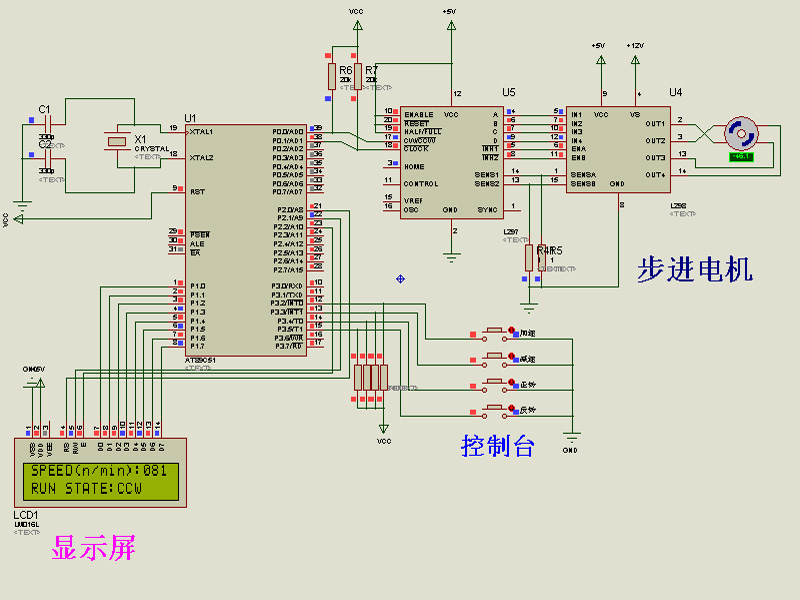##用户名 Email 自动登录 找回密码 密码 立即注册

# 基于51单片机的步进电机调速及下反转控制LCD显示程序+仿真51mcu 发表于 2019-5-10 19:29 | 显示全部楼层 |阅读模式
 基于51单片机的步进电机调速及下反转控制LCD显示，实现步进电机转速调节以及步进电机正反转控制。 全部资料51hei下载地址：基于51单片机的步进电机调速及正反转控制,LCD显示.zip (76.56 KB, 下载次数: 38) 2019-5-10 19:28 上传 点击文件名下载附件 单片机的步进电机调速及正反转控制LCD显示 基于51单片机的步进电机调速及正反转控制LCD显示单片机源程序如下: #include "AT89X51.h" int delay(); void inti_lcd(); void show_lcd(int); void cmd_wr(); void ShowState(); void clock(unsigned int Delay) ; void DoSpeed();       //计算速度 //正转值 #define RIGHT_RUN 1 //反转值 #define LEFT_RUN 0 sbit RS=0xA0; sbit RW=0xA1; sbit E=0xA2; char SpeedChar[]="SPEED(n/min):"; char StateChar[]="RUN STATE:"; char STATE_CW[]="CW"; char STATE_CCW[]="CCW"; char SPEED="050"; unsigned int RunSpeed=50;                //速度 unsigned char RunState=RIGHT_RUN;  //运行状态 main() {                  /*定时器设置*/         TMOD=0x66;            //定时器0，1都为计数方式；方式2；         EA=1;                        //开中断                  TH0=0xff;                //定时器0初值FFH；         TL0=0xff;         ET0=1;         TR0=1;                          TH1=0xff;                //定时器1初值FFH；         TL1=0xff;         ET1=1;         TR1=1;                     IT0=1;                        //脉冲方式         EX0=1;                        //开外部中断0:加速         IT1=1;                        //脉冲方式         EX1=1;                        //开外部中断1:减速     inti_lcd();     DoSpeed();           ShowState();     while(1)     {       clock(RunSpeed);       P0_1=P0_1^0x01;     } } //定时器0中断程序:正转 void t_0(void) interrupt 1 {         RunState=RIGHT_RUN;             P0_0=1;     P1=0x01;     cmd_wr();     ShowState(); } //定时器1中断:反转 void t_1(void) interrupt 3 {         RunState=LEFT_RUN;     P0_0=0;     P1=0x01;     cmd_wr();     ShowState(); } //中断0:加速程序 void SpeedUp() interrupt 0 {           if(RunSpeed>=12)           RunSpeed=RunSpeed-2;        DoSpeed();        P1=0x01;        cmd_wr();        ShowState(); } //中断1:减速程序 void SpeedDowm() interrupt 2 {        if(RunSpeed<=100)         RunSpeed=RunSpeed+2;     DoSpeed();     P1=0x01;     cmd_wr();     ShowState(); } int delay()         //判断LCD是否忙 {        int a; start:     RS=0;     RW=1;     E=0;     for(a=0;a<2;a++);     E=1;     P1=0xff;     if(P1_7==0)        return 0;     else                goto start; } void inti_lcd()  //设置LCD方式 {    P1=0x38;    cmd_wr();    delay();    P1=0x01;     //清除    cmd_wr();    delay();    P1=0x0f;    cmd_wr();    delay();    P1=0x06;    cmd_wr();    delay();    P1=0x0c;    cmd_wr();    delay(); } void cmd_wr()           //写控制字 {    RS=0;    RW=0;    E=0;    E=1; } void show_lcd(int i)   //LCD显示子程序 {      P1=i;    RS=1;    RW=0;    E=0;    E=1; } void ShowState()    //显示状态与速度 {     int i=0;     while(SpeedChar[i]!='\0')     {        delay();        show_lcd(SpeedChar[i]);        i++;     }     delay();     P1=0x80 | 0x0d;     cmd_wr();     i=0;     while(SPEED[i]!='\0')     {        delay();        show_lcd(SPEED[i]);        i++;     }     delay();     P1=0xC0;     cmd_wr();     i=0;     while(StateChar[i]!='\0')     {        delay();        show_lcd(StateChar[i]);        i++;     }     delay();     P1=0xC0 | 0x0A;     cmd_wr();     i=0;     if(RunState==RIGHT_RUN)         while(STATE_CW[i]!='\0')         {           delay();           show_lcd(STATE_CW[i]);           i++;         }     else        while(STATE_CCW[i]!='\0')         {           delay();           show_lcd(STATE_CCW[i]);           i++;         } } void clock(unsigned int Delay)   //1ms延时程序 {  unsigned int i;    for(;Delay>0;Delay--)     for(i=0;i<124;i++); } void DoSpeed() {     SPEED=(1000*6/RunSpeed/100)+48;     SPEED=1000*6/RunSpeed%100/10+48;     SPEED=1000*6/RunSpeed%10+48; }复制代码

### 评分

 本版积分规则 回帖并转播 回帖后跳转到最后一页

Powered by 单片机教程网